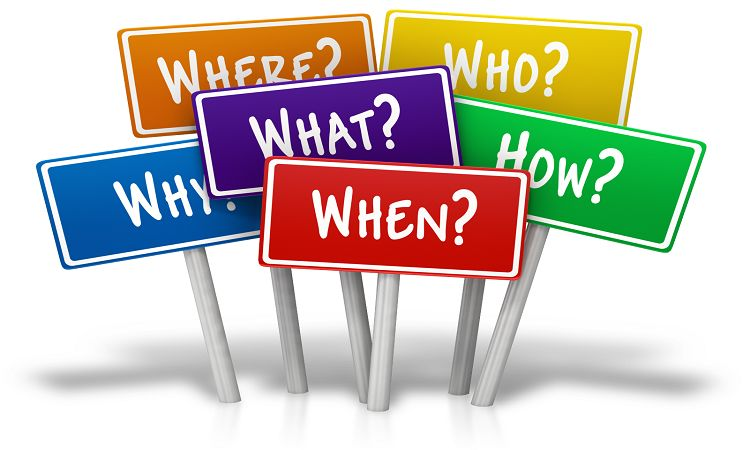# How many equal angles has a scalene triangle ? The answer is

Question: How many equal angles has a scalene triangle